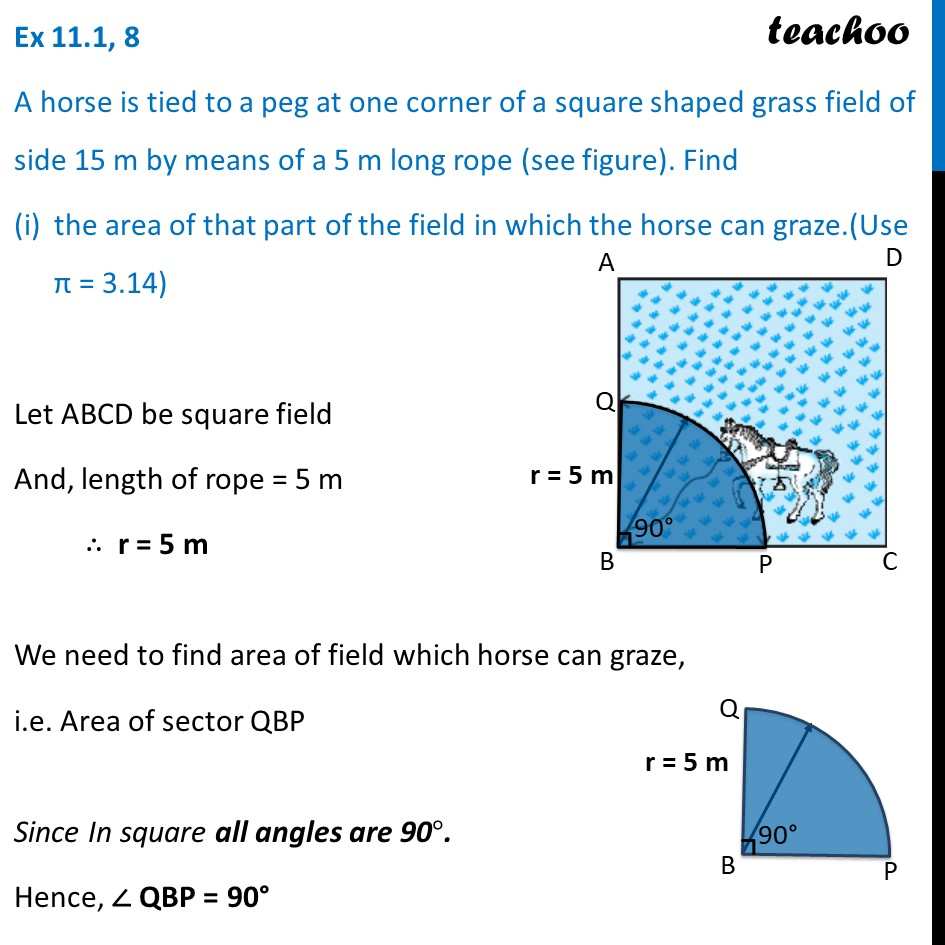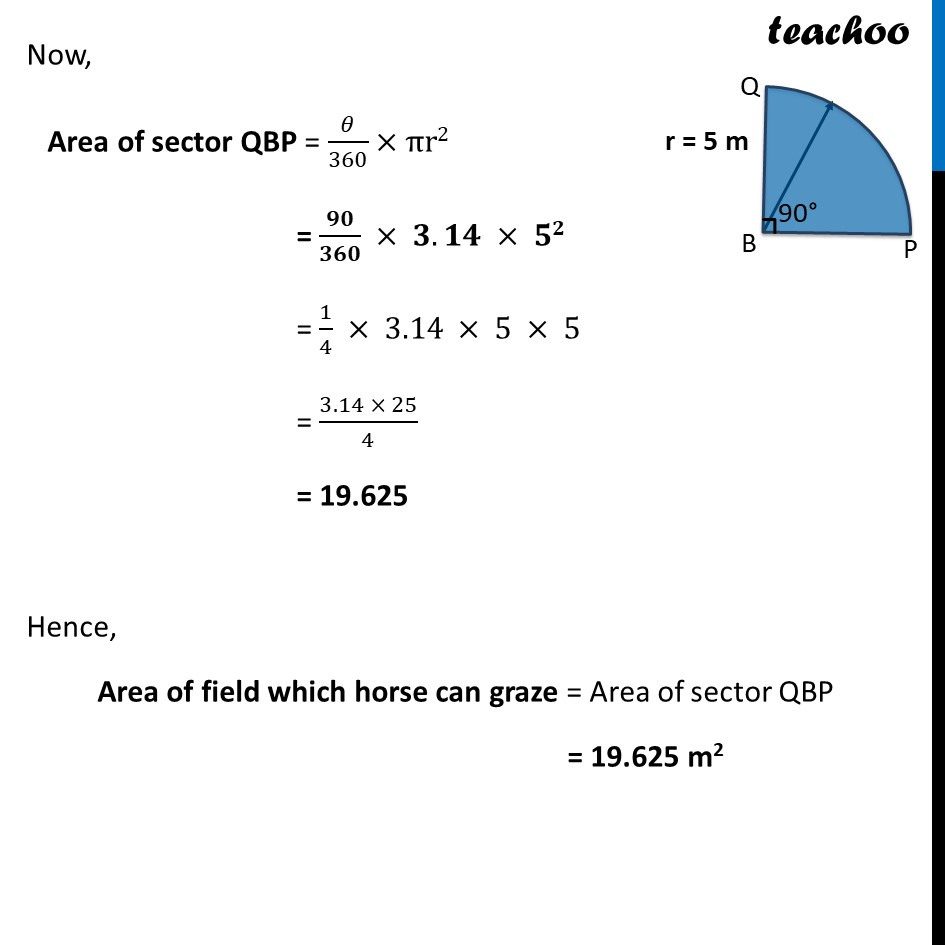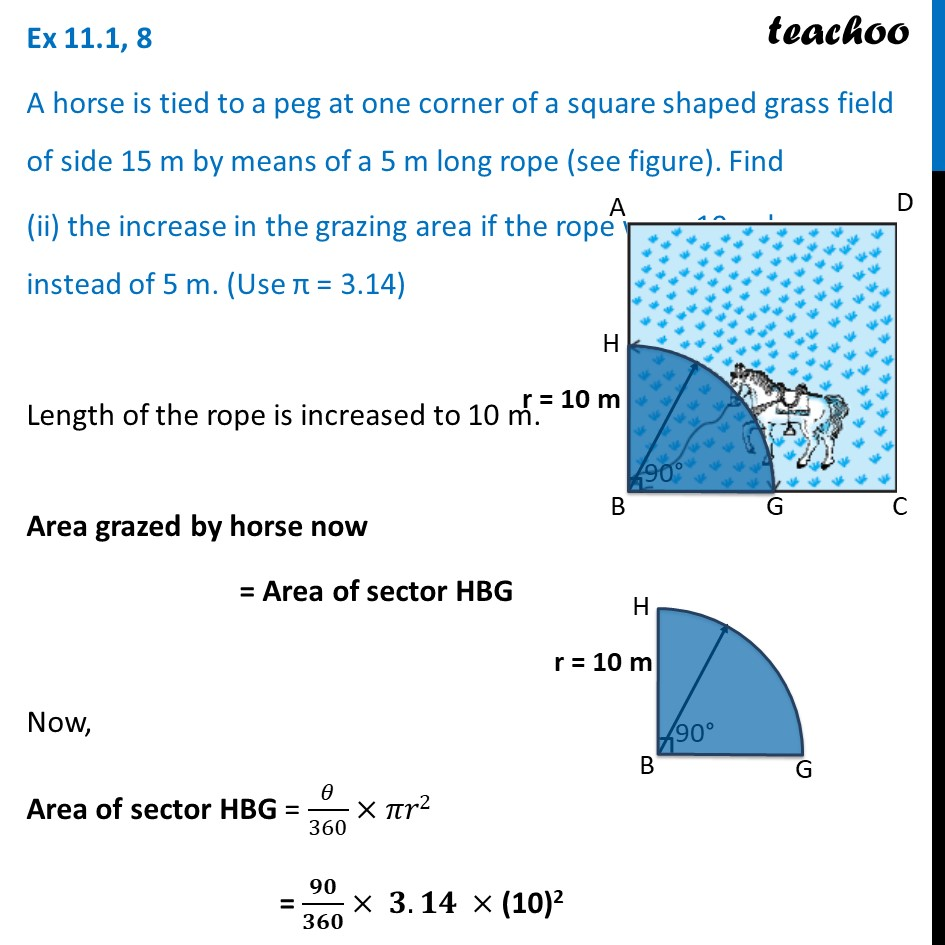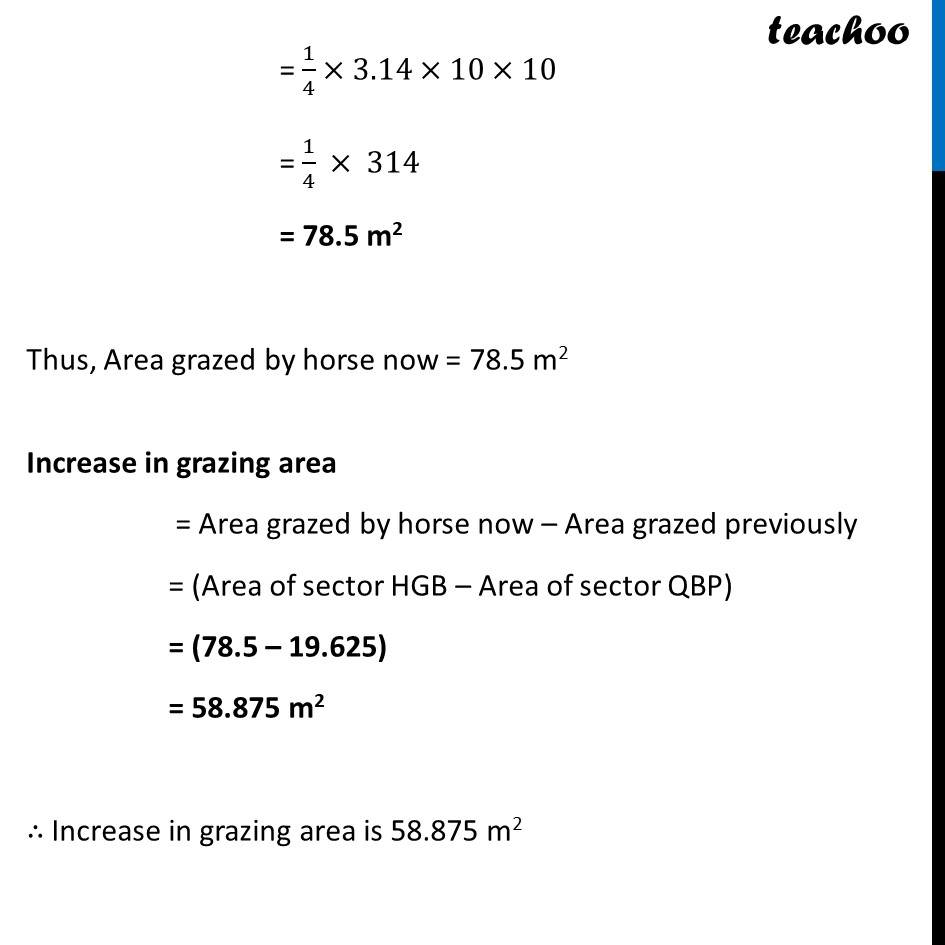Area of sector of circle

Chapter 11 Class 10 Areas related to Circles
Concept wiseLearn in your speed, with individual attention - Teachoo Maths 1-on-1 Class

### Transcript

Ex 11.1, 8 A horse is tied to a peg at one corner of a square shaped grass field of side 15 m by means of a 5 m long rope (see figure). Find the area of that part of the field in which the horse can graze.(Use π = 3.14) Let ABCD be square field And, length of rope = 5 m ∴ r = 5 m We need to find area of field which horse can graze, i.e. Area of sector QBP Since In square all angles are 90°. Hence, ∠ QBP = 90° Now, Area of sector QBP = 𝜃/360×πr2 = 𝟗𝟎/𝟑𝟔𝟎 × 𝟑.𝟏𝟒 × 𝟓𝟐 = 1/4 × 3.14 × 5 × 5 = (3.14 × 25)/4 = 19.625 Hence, Area of field which horse can graze = Area of sector QBP = 19.625 m2 Ex 11.1, 8 A horse is tied to a peg at one corner of a square shaped grass field of side 15 m by means of a 5 m long rope (see figure). Find (ii) the increase in the grazing area if the rope were 10 m long instead of 5 m. (Use π = 3.14) Length of the rope is increased to 10 m. Area grazed by horse now = Area of sector HBG Now, Area of sector HBG = 𝜃/360×𝜋𝑟2 = 𝟗𝟎/𝟑𝟔𝟎× 𝟑.𝟏𝟒 × (10)2 = 1/4×3.14×10×10 = 1/4 × 314 = 78.5 m2 Thus, Area grazed by horse now = 78.5 m2 Increase in grazing area = Area grazed by horse now – Area grazed previously = (Area of sector HGB – Area of sector QBP) = (78.5 – 19.625) = 58.875 m2 ∴ Increase in grazing area is 58.875 m2